# 1st PUC Economics Question Bank Chapter 8 Index Numbers

Students can Download Economics Chapter 8 Index Numbers Questions and Answers, Notes Pdf, 1st PUC Economics Question Bank with Answers helps you to revise the complete Karnataka State Board Syllabus and score more marks in your examinations.

## Karnataka 1st PUC Economics Question Bank Chapter 8 Index Numbers

### 1st PUC Economics Index Numbers TextBook Questions and Answers

Question 1.
An index number which accounts for the relative importance of the items is known as:

1. Weighted index
2. Simple aggregative index
3. Simple average of relative.

1. An Index Number which accounts for the relative importance of the items is known as weighted index.

Question 2.
In most of the weighted index numbers the weight pertains to :

1. base year
2. current year
3. both base and current year

1. In most of the weighted index number, the weight pertains to the base year.

Question 3.
The impact of change in the price of a commodity’ with little weight in the index will be:

1. small
2. large
3. uncertain

Question 4.
A consumer price index measures changes in:

1. retail prices
2. whole sale prices
3. Production prices

1. A consumer price index measures changes in retail prices.Question 5.
The item having the highest weight in consumer price index for industrial workers is :

1. food
2. housing
3. clothing

The item having the highest weight in consumer price index for industrial workers is food.

Question 6.
In general, inflation is calculated by using:

1. Wholesale price index
2. consumer price index
3. producer’s price index.

In general inflation is calculated by using the wholesale price index.

Question 7.
Why do we need an index number?
We need index number for measuring changes in the magnitude of a group of related variables.Question 8.
What are the desirable properties of the base year?
Desirable properties of the base year:

1. As far as possible, the base year should be a normal year i.e. it should be the one without ups and downs.
2. Extreme values should not be selected as base period.
3. The period should also not belong to too far in the past.
4. The base period for any index number is updated.

Question 9.
Why is it essential to have different CPI’s for different categories of consumers?
It is essential to name different CPI’s for different categories of consumers as the cost of living of different categories of consumers differ.

Question 10.
What does a consumer price index for industrial workers measure?
A consumer price index for industrial workers measure general inflation.

Question 11.
What is the difference between a Price Index and a Quantity Index? (11)
A Price index measure changes in prices between two Points of time whereas a quantity index measures changes in the volume of goods produce.

Question 12.
Is the change in any price reflected in Price index number? (12)
The change in any price is not reflected in price index number. Price index only shows the changes in the price of goods which are included in the construction of a particular index.Question 13.
Can the CPI for urban non – manual employees represent the changes in the cost of living of the president of India?
Yes, the CPI number for urban non – manual employees represents the changes in the cost of living of the president of India, Because he is also non – manual employee.

Question 14.
Try to list the important items of consumption in your family? (16)
Wheat, Rice, Toothpaste, pulses, clothing, petroleum, housing, means of entertainment such as T.V. transistor, Radio, telephone, mobile, vehicle, books, stationery.

Question 15.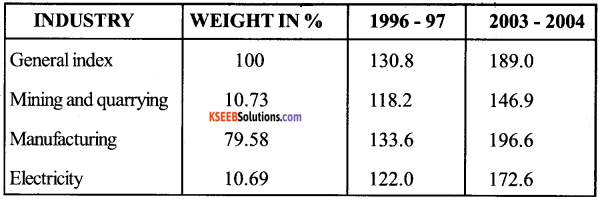1. The table shows that the growth performance of the broad industrial categories differ. The general index represents the average performances of these categories.
2. There is about 45% rise in general index.
3. There has been a comparatively lower performance of mining and quarrying It is about 25%.
4. The comparatively low performance of mining and quarrying has not been able to push down the general index due to high increase in manufacturing index and due to maximum weight given to this sector.

Question 16.
Try to list the important items of consumption in your family.
Wheat, rice, toothpaste, pulses, clothing, petroleum, housing, means of entertainment such as T.V., transistor, radio, telephone, mobile, vehicle, books, stationery.

Question 17.
If the salary of a person in the base year is Rs. 4000 per annum and the current year is Rs. 6000. By how much should his salary rise to maintain the same standard of living if the CPI is Rs. 4000.
Price Index of the Base year = 100
Current year salary = Rs. 6,000
Consumer price index of the current year = 400
His salary should rise to Rs. 16000 to maintain the same standard of living.
$$\frac{\text { Current Year CPI }}{\text { Base Year CPI }}$$ × Base year Salary = $$\frac{400}{100}$$ × 4000 = Rs. 16,000

Thus, there should be a rise of Rs. 10,000 ( 16000-6000) in the salary of that person to maintain the same standard of living.Question 18.
The consumer price index for June 2005 was 125. The food index was 120 and that of other items 135. What is the percentage of the total weight given to food?
Suppose the percentage of weight given to food = x
In this case percentage of weight given to other items would be = (100 – x) or
12500 = 120x + 13500 – 135x
15x = 13500 – 12500=1000
x = $$\frac{1000}{15}$$= 66.66%
Hence, percentage of the total weight given to food = 66.66.

Question 19.
An enquiry into the budgets of the middle class families in a certain city gave the following information.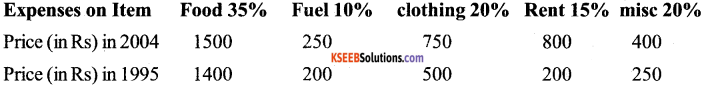What is the cost of living index of 2004 as compared with 1995?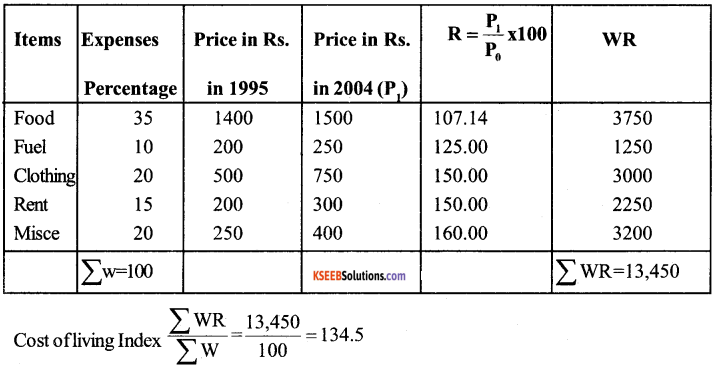Question 20.
Record the only expenditure, quantities bought and prices paid per unit of the daily purchases of your family for two weeks. How has the price change affected you family?
The students are advised that they should enquire of their parents about the daily expenditure quantities bought and prices paid per unit of daily purchases of their family for two weeks. They will come to know that the rising prices of goods have effected that family very much;
For example: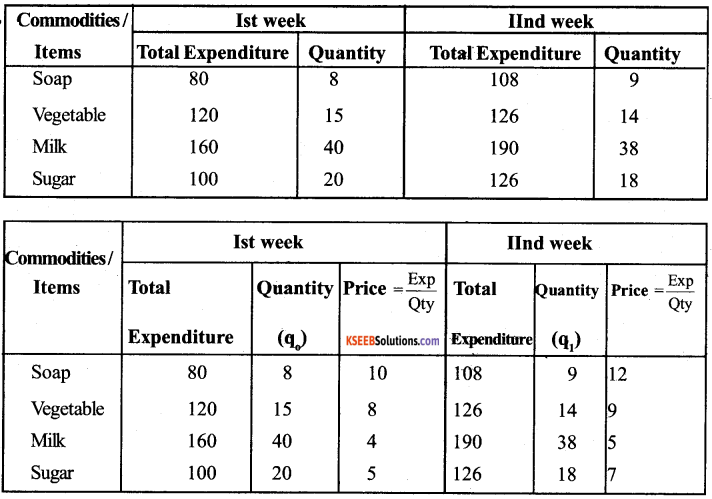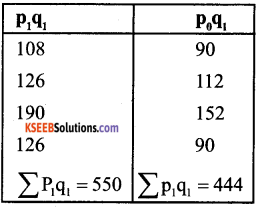Σ p01 = $$\frac{\sum p_{1} q_{1}}{\sum p_{0} q_{1}}=\frac{550}{444}$$ × 100 = 1.2387 × 100 = 123.87

Price index of the 22nd week P01 = 123.87, which means that price increased by 23.87% compared to 1st week. Family has to increase its expenditure by 23.87% for maintaining the same level of living of the 1st week.

1st PUC Economics Index Numbers Very Short Answer Type Questions

Question 1.
Define index number?
An index number is a statistical measure designed to show change in a variable or group of related variables with respect to time, geographic location or other characteristics.

Question 2.
What is the difference between simple index number and weighted index number?
In the simple index number all items of the series are treated as of equal importance. In the weighted index, weights are accorded to different items-depending on their relative importance.Question 3.
What should be the base year like?
The base year should be the year of economics stability. It should not to be too distant from the current year.

Question 4.
Define prime index number?
Price index number is that number which measures and permits comparison of the price of certain goods.

Question 5.
State two types of price index number

1. Consumer price index or cost of living index number.
2. Wholesale price index number.

Question 6.
Name the consumer groups for which consumers price index number is computed in India?

1. Industrial workers.
2. Urban – non manual employees.
3. Agricultural labourers.

Question 7.
What is the other name of the Consumer? Price Index?
The other name of the consumer price index is price deflater of income.

Question 8.
What do ‘inflation’ and ‘deflation’ refer to?
‘Inflation’ refers to increase in prices and ‘deflation’ refers to decrease in prices.Question 9.
Write down any two characteristics of index numbers?

1. Index numbers are expressed in percentage.
2. They are specialised type of average.

Question 10.
What does consumer price index measure?
Consumer price index measures the average changes in retail prices at which the consumers buy goods and services in the market at a given price.

Question 11.
Which price index is an indicator of change in the ‘general price level’
The wholesale price index is an indicator of change in general price level.

Question 12.
What is the base year of the new series of wholesale price index numbers published , by the govt, of india?
1993 – 1994

Question 13.
Write down the formula for calculating the inflation rate.
Inflation rate = $$\frac{x_{1}-x_{1}-1}{x_{1}-1}$$ × 100

Question 14.
Which index number is used to calculate the purchasing power of money and real wages?
Consumer price index (CPI)Question 15.
Write down the formula for calculating purchasing power of money?
Purchase Power of Money = $$\frac{1}{\cos t \text { of living index }}$$

Question 16.
Calculate the purchasing power of one rupee when the cost of living index is 526 (1932 =100)
Purchasing power of one rupee = $$\frac{1}{\cos t \text { of living index }}$$

Question 17.
Write down the formula for calculating real wages.
Real wages = $$\frac{\text { money wages }}{\text { cost of living index }}$$

Question 18.
What will be the real wage of the consumer if his money wage is Rs. 10,000 and the cost of living index in 526?
Real wages = $$\frac{\text { money wages }}{\text { cost of living index }}$$ × 100

Question 19.
If the salary of a person in the base year was Rs. 3000 per annum and his current year salary is 10,000 by how much should his salary rise to maintain the same standard of living if CPI is 526.
To maintain the same strandard of living a man’s salary should be Rs. 1570
$$\left(\frac{3000}{100} \times 526\right)$$
so the salary should be raised to 15780, This salary should be added by Rs. 5,780

Question 20.
The CPI (1982 = 100) is Rs. 526 in Jan 2005. Calculate the equivalent of a rupee in Jan 2005
Equivalent of a rupees. It means that the rupee in Jan 2005 was worth 19 paise in 1982. In other worths 19 paise in 1982 in worth Rs. 1 in 2005 That is 526 times.

Question 21.
Write down the widely used index numbers?
Widely used index numbers are whole sales price index number, consumer price index, index for industrial production, agricultural production index and sensex.Question 22.
What is the other name of Consumer Price Index (CPI)?
The other name of the Consumer Price index is the cost of living index.

Question 23.
In how many group are the industries grouped for construction of index of industrial production in India Name them.
Industries are grouped into three categories

1. Mining
2. Manufacturing and
3. Electricity for constructing index of Industrial production of India.

Question 24.
What are the various methods of construction index number?

1. Simple aggregative method.
2. simple average of price relative method
3. Weighted Aggregative method and
4. Weighted average of Price relative method.

Question 25.
What does sensex stand for?
Sensex stands for Bombay stock exchange sensitive Index with 1978 – 79 as base.

Question 26.
What is an independent variable?
An independent variable is that variable which is not dependent on any other values. Its value is free.

Question 27.
What is dependent variable?
A dependent variable is that variable whose value depends on the value of an independent values.

1st PUC Economics Index Numbers Short Answer Type Questions

Question 1.
Define Price Index?
Index number is a statistical measure showing percentage or relative changes in variables like prices, production, exports etc.
According to Spiegel:
An Index number is a statistical measure designed to show changes in a variable or group of related variables with respect to time, geographic location or other characteristics.

Question 2.
Give utility of index numbers?
Utility of index numbers

1. Index numbers are helpful to policymakers.
2. Index numbers simplify the facts.
3. Comparative study become easy.
4. Index number help us to study the general trend.
5. They are helpful in determination of real change in income.

Question 3.
What are the limitations of index numbers?
In the construction of index numbers, there are some practical difficulties and theoretical limitations.

1. Index numbers are not fully true. They simply indicate arithmetical tendency of the temporal change in the variable.
2. Index numbers do not help in international comparison.
3. Index numbers are prepared with certain specific objective.
4. It is difficult to collect retail prices so index numbers based on wholesale prices may be misleading.Question 4.
What are the consideration underlying the choice of base period in construction of an index number?
Basic consideration underlying the choice of base period.

1. The base period should be a normal period. Abnormal periods like period of war or floods etc. should not be selected as a base year.
2. The base period should be neither too short nor too long.
3. It should not be the period for which actual data are not available.
4. It should not be the too far back in the past.

Question 5.
Discuss the simple Aggregative Price Index. What are its limitations?
Simple Aggregative Price Index: A Simple Aggregative Price Index is defined as.
P01 = $$\frac{\sum P_{11}}{P_{10}}$$ × 100
Which is the sum of prices of commodities in the current period ‘I’ expressed as the sum of prices in the base year ‘ o ’
Limitations:

1. It has limited applicability.
2. No weightage is given to the relative importance of items.
3. Index is influenced by the itmes with the large unit prices.

Question 6.
Write down the uses of the wholesale Price Indiex numbers?
Uses of the Wholesale Price Index numbers:

1. The time series of wholesale Price index numbers can be used to forecast future prices.
2. With the help of wholesale price index number, we can estimate the future demand and supply situation.
3. It is used to measure the rate of inflation.
4. It can be used to eliminate the effect of change in price on aggregates such as national income, capital formation etc.

Question 7.
Discuss the “weighted” and “unweighted” Index of Prices?
“Weighted ” Index of Prices: In order to allow a dequate importance to different commodities in a composite index, we assign suitable weights to them. The weighted index number is simply the weighted arithmetic mean of Price relatives defined as
P01 = Σ W1 $$\frac{P_{11}}{P_{10}}$$
Where the weights W1 W2….. W11 are such the Σ W1 = 1Question 8.
The consumer Price index for june, 2005 was 125. The food index was 120 and that of other items 135. What is the Percentage of the total weight given to food?
Suppose the Percentage of weight given to food = x
In this case Percentage of weight given to other items would be = (100 – x)
Hence,
125 = $$\frac{(\mathrm{X} \times 120)+(100-\mathrm{x}) \times 135}{100}$$
or 12,500 = 12x + 13,500 – 135x
or 15x = 13,500 – 12,500 = 1,000
X = $$\frac{1,000}{15}$$ = 66.66%
Hence, Percentage of the total weight given to food = 66.66.

Question 9.
Many difficulties are faced to Prepare index number. Explain any two of them?
Many Problems are faced while constructing an index number. Two of them are as follows:
1. Selection of base year:
The first problem which arises while preparing Index number is the section of base year. Which year should be selected as a base? While selecting a base year, we should remember that it should be a normal period. It should not be either too short or too long. It should be free from all sorts of abnormalities or irregular fluctuations.

2. Selection of items:
We should also be careful in selecting the items to be included in the index number. Items selected should be relevant to the purpose of the index. The items should be standardized which are easy to describe and understand. Moreover, the total number of commodities selected for the index should be neither too small nor too large.

Question 10.
Write down the usefulness of Consumer Price index or cost of living index?

1. It is helpful in wage negotiation.
2. It is helpful in the formation of income Policy, Price Policy taxation and general economic policy formulation.
3. It is used in calculating the purchasing power of money.
4. With the help of CPI, we can calculate the real wages.

Question 11.
Record the only expenditure, quantities bought and prices paid per unit of the daily purchases of your family for two weeks. How has the Price Change affected your family.
The students are advised that they should enquire their parents about the daily expenditure. Quantities bought and Prices paid per unit of daily purchase of their family for two weeks, they will come to know that the rising prices of goods have effected that family very much.

Question 12.
Show the methods of constructing index numbers with the help of a diagram?
i) Method of constructing simple index numbers:

• Simple aggregative method.
• Simple average of price relative method.

ii) Methods of constructing weighted index numbers.

• Weighted aggregative method.
• Weighted average of Price relative method.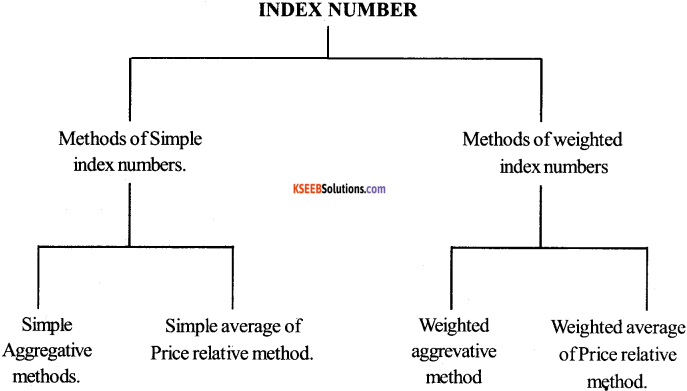1st PUC Economics Index Numbers Long Answer Type Questions

Question 1.
Write down the features of consumer Price index number.
Features of CPI. Following are main features of CPI:
1. In India three CPI’s are Constructed.

• CPI for industrial workers.
• CPI for urban non-manual employees and
• CPI for agricultural labourers.

2. The base year for CPI for industrial workers, CPI for urban non – manual employees and CPI for agricultural labourers are 1982,1984-85 and 1986 respectively.

3. They are routinely calculated every month to analyse the impact of changes in the retail Price on the cost of living of these three broad categories of consumers.

5. The Central Statistical Organisation publishes the CPI for urban non – manual employees.

6. The items shown in the table are taked on while calculating CPI for industrial workers form the table we come to know that food has the largest weight.

CPI for industrial workers.

 Major Crops Weight in percentage Food 57.00 Pan, Supari, Tabacco etc 3.15 Fuel and light 6.28 Housing 8.67 Clothing, bedding and footwear 8.54 Misc group 16.36 General 100.00Question 2.
Explain the importance of stock statistics in Economy.
Importance of Index Numbers:
Index Numbers have a great importance in Statistics.
They are used in the various fields of economics. Such as production, consumption, Price etc

With the help of Statistics, economic conditions can be studied and forecast can be made. They are called the “Economic Barometer” Their importance can be judged with the following facts.

1. Calculation of Price levels:
Index Numbers help in calculating levels of various items.

Index numbers are very helpful of Government in taking important decisions, such as granting dearness allowances to the employees.

They also help us in making comparative study of the changes occurring in the economy.

4. Helpful do insurance companies and Banking Institution. Index numbers help the insurance companies and banking institutions in determining of Premium – rates and interests respectively.

5. Helpful in assesing the development of an economy with the help of statistics, the development of an economy can be assessed.

Question 3.
Calculate the unweighted index of prices for the data given below.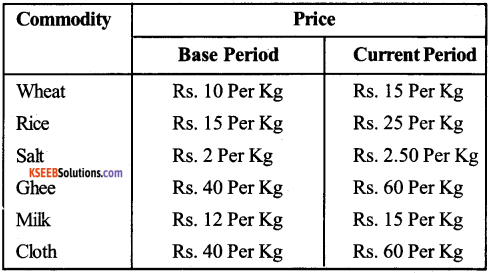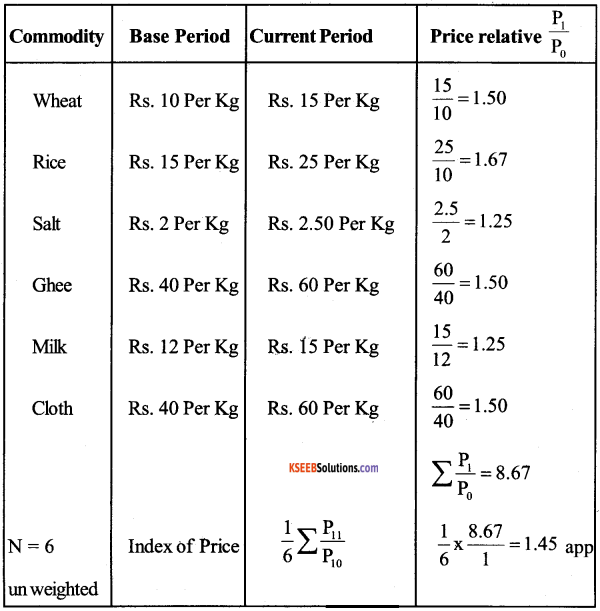Question 4.
Calculate weighted index of Price of the following data.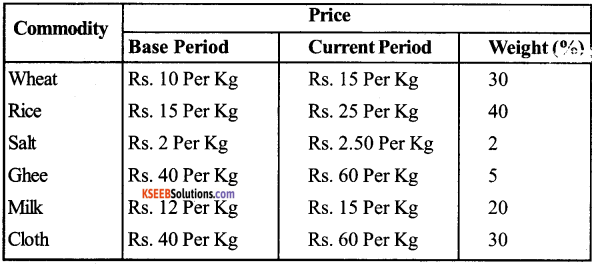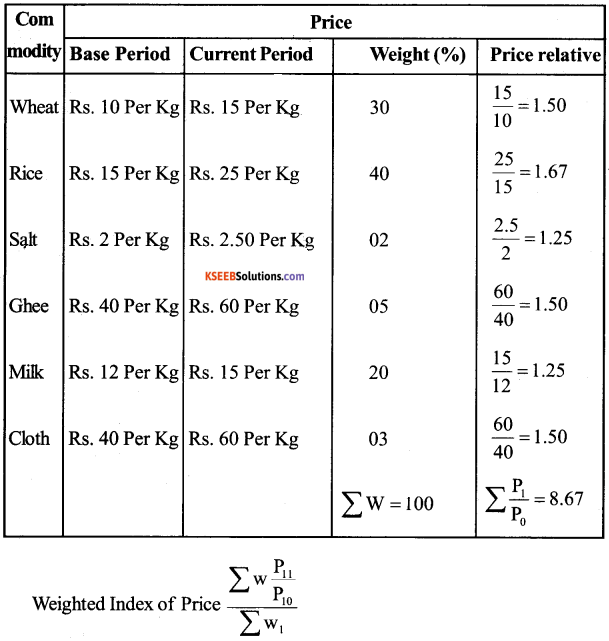= 30 × 1.5 + $$\frac{40 \times 1.67+5 \times 1.5}{10}$$ + 20 × 1.25 × 1.50 = 1.51 × 100 = 15.1 %Question 5.
Calculate index numbers for the period from 1995 to 2001, taking 1994 as base year: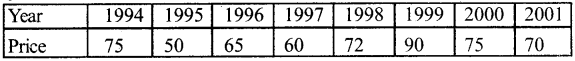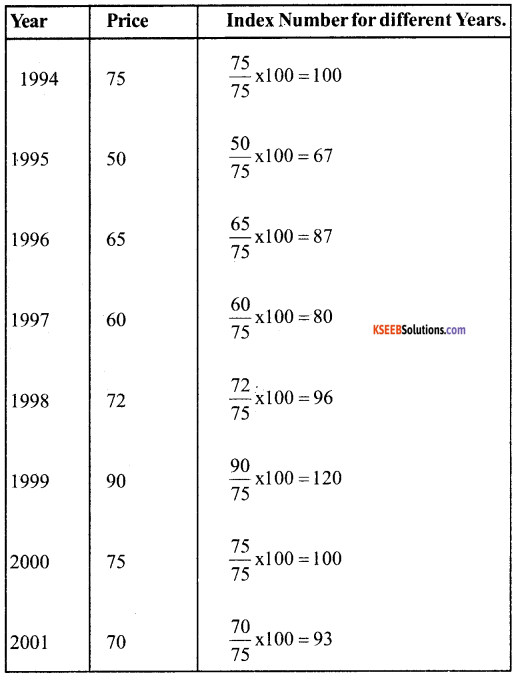Question 6.
Calculate index numbers for the different years taking 1984 as the base year: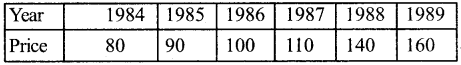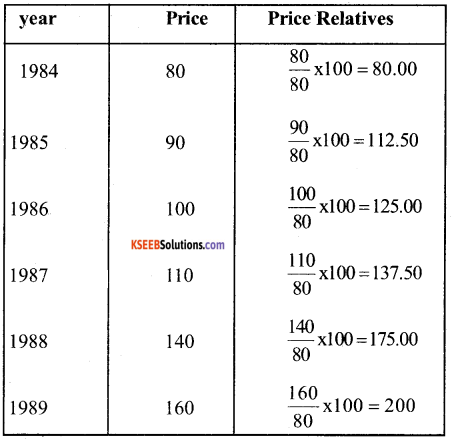Question 7.
Calculate the cost of living index from the following data: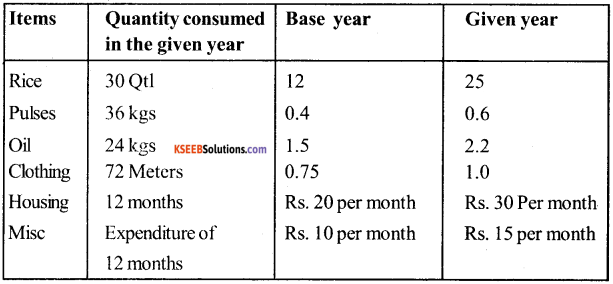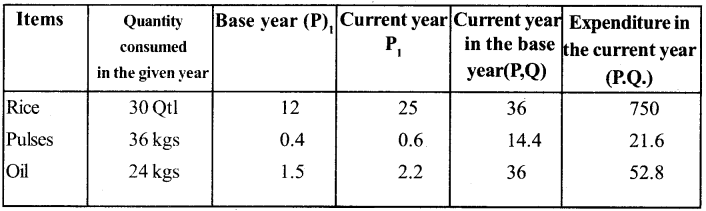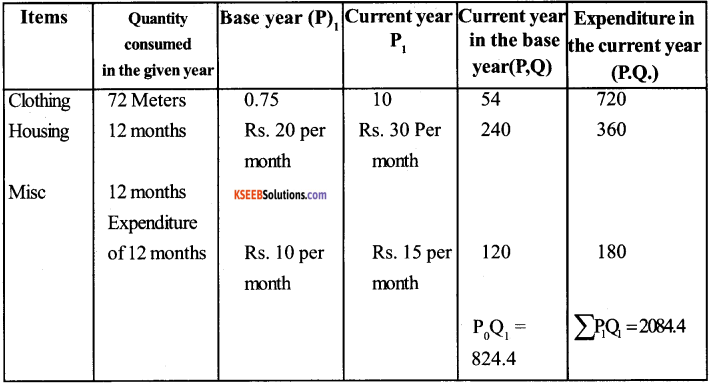Cost of living Index number
= $$\frac{\sum P_{1} Q_{1}}{\sum P_{0} Q_{1}}$$ × 100 = $$=\frac{2084.4}{824.4}$$ × 100 = 252.2Question 8.
Construct consumer Pice index number with the help of following date. The weight of these items are 35, 10, 20, 15, 20 respectively.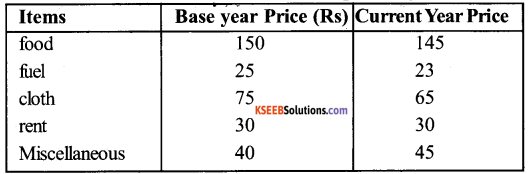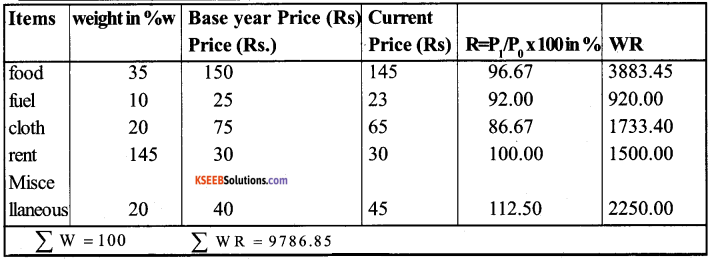Question 9.
The monthly per captia expenditure incurred by workers for an industrial centre during 1980 and 2005 on the following items are 75,10,6 and 4 respectively. Prepare a weighted index number for cost of living for 2005 with 1980 as the base.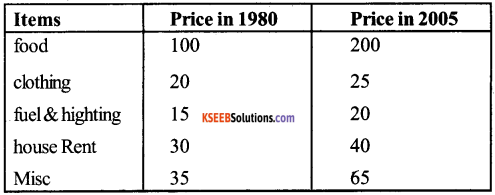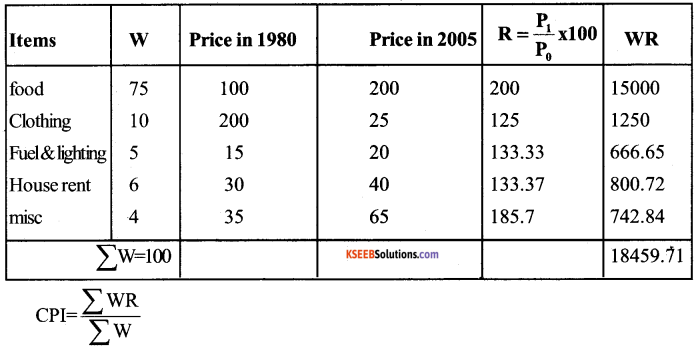= $$\frac{18459.71}{1000}$$ = 184.60

Question 10.
Calculate the Simple aggrevative price inde number with the help of following: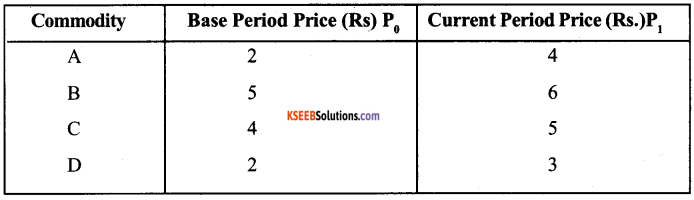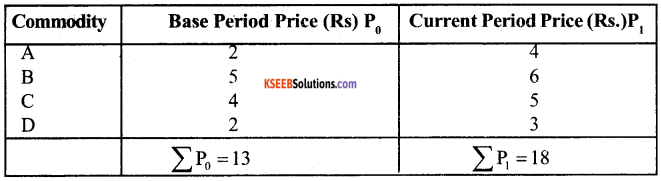P01 $$\frac{\sum P_{1}}{\sum P_{0}}$$ × 100 = $$\frac{18}{13}$$ × 100 = 138.46Question 11.
Calculate Weighted aggregative price index from the following table using

1. Laspeyre’s method &
2. Laspeyre’s Method.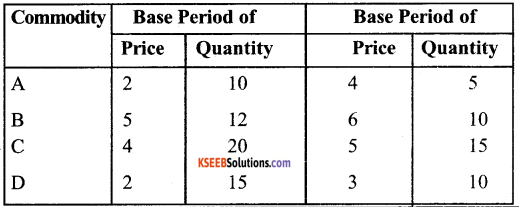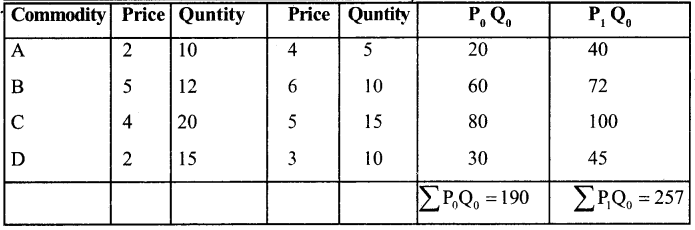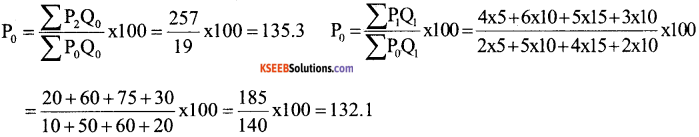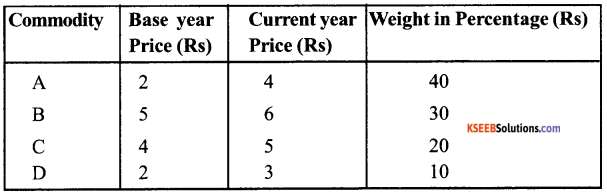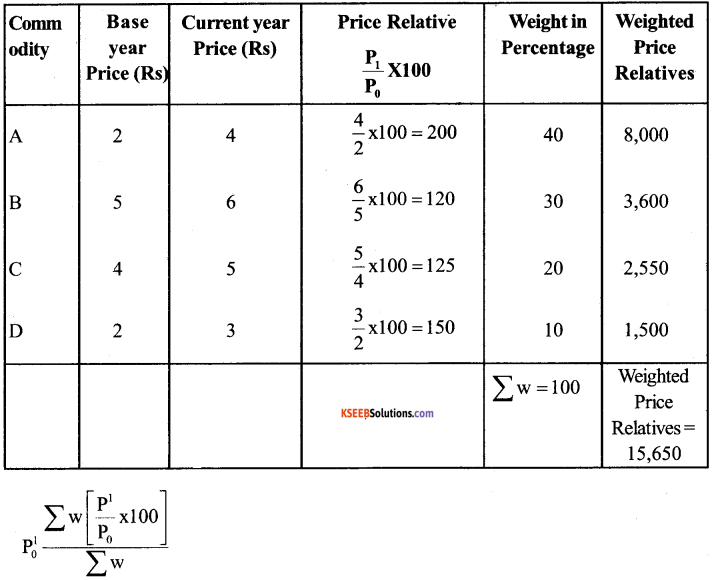= $$\frac{15650}{100}$$